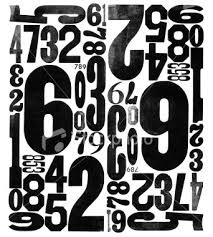# This is fun!While doing some operations i came across a number with the following property. a^{b} X c^{d} =abcd. Which is the number abcd satisfying the above property. (Remember abcd means the positioning of the numbers a,b,c,d and not multiplication)

×